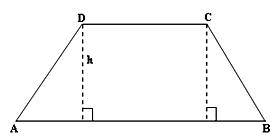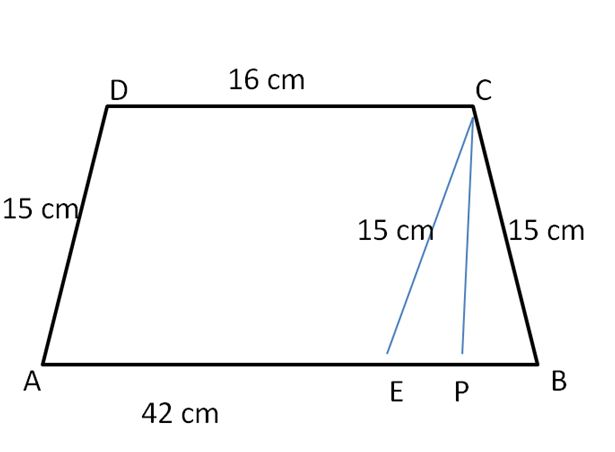# Area of a Trapezium- Definition, Formula, Properties, Examples

The trapezium is a quadrilateral that has four sides and one set of parallel sides. The area of trapezium is the region covered by the solid in a two-dimensional plane. Other types of quadrilaterals are parallelogram, rectangle, square, and rhombus. Find the definition, formula, and solved examples on the trapezium area.

## Trapezium Definition

The trapezium is a flat closed shape having 4 straight sides with one pair of parallel sides. The parallel sides are called the bases and the non-parallel sides are called the legs. The different types of the trapezium are isosceles trapezium, scalene trapezium, and right trapezium. Some of the properties of the trapezium are along the lines:

• Every trapezium has exactly one pair of parallel and opposite sides.
• The diagonals intersect each other.
• The non-parallel sides in trapezium are unequal except in isosceles trapezium.
• The line that joins the mid-points of the non-parallel sides is always parallel to the bases or parallel sides which is equal to half of the sum of parallel sides.
• In isosceles trapezium, the non parallel sides are congruent
• The sum of interior angles is equal to 360°.
• The sum of two adjacent angles is 180°.

### Area of a Trapezium

The trapezium area is the region covered by the trapezium in a two-dimensional plane. It can be measured by taking the average of two bases and multiplied the result by altitude. Therefore, the area of the trapezium formula is given as,

Trapezium Area A = h(a + b)/2 square units

Where,

a, b are the bases

h is the height or altitude

Area of an isosceles Trapezium = 1/2 [√(c² – (a – b)²) (a + b)]

Where,

a = AB, b = CD, c = AD

The perimeter of trapezium P = a + b + c + d units

Perimeter of the Isosceles Trapezium = a + b + 2c unitsProve that:

Area of trapezium ABCD = 1/2[AD + BC] h

Area of trapezium ABCD = area (∆DFA) + area (rectangle DFEC) + area (∆CEB)

= (¹/₂ × AF × DF) + (FE × DF) + (¹/₂ × EB × CE)

= (¹/₂ × AF × h) + (FE × h) + (¹/₂ × EB × h)

= h/2 x (AF + FE + EB)

= h/2 × (AB + FE)

= h/2 x (AB + DC)

= ¹/₂ × (sum of parallel sides) × (distance between them)

Therefore, The area of a trapezium = ¹/₂ × (sum of parallel sides) × (distance between them)

### How to find the Trapezium Area?

Follow the steps to calculate the Area of the Trapezium by referring to the below steps. They are as such

• Observe the question carefully and make a note of known parameters.
• Find either height, base sides, or sum of parallel sides, the distance between the parallel sides.
• Substitute these values in the formula to get the area.

### Area of a Trapezium Questions and Answers

Example 1.

In a trapezium, the measurement of one parallel side two more than the other parallel side and the height is 6 cm. The area of the trapezium is 216 cm². Find the lengths of the two parallel sides?

Solution:

Let a, b are the parallel sides of the trapezium.

According to the question,

One parallel side is two more than the other parallel side

a = b + 2

Area of the trapezium = 216 cm²

½(a + b)h = 216

Substitute a = b + 2, h = 6 cm in above equation.

½(b + 2 + b)4 = 216

2(2b + 2) = 216

2b + 2 = 216/2

2b + 2 = 108

2b = 108 – 2

2b = 106

b = 106/2

b = 53

Put b = 53 in a = b + 2

a = 53 + 2 = 55

So, the lengths of the two parallel sides are 55 cm, 53 cm.

Example 2.

The area of a trapezium is 148 cm² and its height is 12 cm. If one of the parallel sides is double of the other, find the two parallel sides?

Solution:

Given that,

Area of the trapezium = 148 cm²

Height = 12 cm

Let one side of the trapezium is a, then another parallel side is b.

As per the question,

One of the parallel sides is double of the other.

a = 2b

Area of the trapezium = 148 cm²

½(a + b)h = 148

Substitute a = 2b, h = 12 in above equation.

½(2b + b) x 12 = 148

3b x 6 = 148

18b = 148

b = 148/18

b = 8.2

Put b = 8.2 in a = 2b

a = 8.2 x 2

a = 16.4

Therefore, the two parallel sides of length are 16.4 cm, 8.2 cm.

Example 3.

Find the area of a trapezium whose parallel sides are AB = 42 cm, CD = 16 cm, and non parallel side AD = 15 cm, BC = 15 cm?Solution:

In trapezium ABCD,

CE = 15 cm

CD = 16 cm, AE = 16 cm

EB = AB – AE = 42 – 16 = 26 cm

Now, in ∆ EBC

S = (15 + 15 + 26)/2

S = 56/2 = 28

Area of triangle = √(s(s – a) (s – b) (s – c))

= √(28 (28 – 15) (28 – 15) (28 – 26))

= √(28 (13) (13) (2))

= √(9464) = 97.2 cm²

Area of ∆EBC = 1/2 × EB × CP

97.2 = 1/2 × 26 × CP

CP x 13 = 97.2

CP = 97.2/13 = 7.48 cm

Therefore, h = 7.48 cm

Area of trapezium = 1/2(p₁ + p₂) × h

= 1/2(58) × 7.48

= 29 x 7.48 = 216.92 cm²

Therefore, area of a trapezium is 216.92 cm².

Example 4.

The length of the parallel sides of a trapezium is in the ratio 5: 8 and the distance between them is 18 cm. If the area of the trapezium is 256 cm², find the length of the parallel sides?

Solution:

Given that,

The ratio of parallel sides of the trapezium is 5: 8.

The distance between parallel sides = 18 cm

Area of the trapezium = 256 cm²

Let the common ratio be x,

Then, parallel sides are 5x, 8x.

Area of trapezium = 1/2 (p₁ + p₂) h

256 = 1/2 (5x + 8x) x 18

256 = 9(13x)

256 = 117x

x = 256/117

x = 2.188

5x = 5 x 2.188 = 10.94, 8x = 8 x 2.188 = 17.5

Therefore, the length of parallel sides area are 10.94 cm and 17.5 cm.

### FAQs on Area of a Trapezium

1. What is the formula for the Area of a trapezium?

Area of trapezium = ½ x Sum of parallel sides x Distance between the parallel sides

Trapezium area = ½ x (a+b) x h

2. What are the applications of a trapezium?

It is used in various physics computations and mathematical calculations.

3. What are the properties of Trapezium?
The trapezium has 4 unequal sides, 2 are parallel and 2 are nonparallel. The sum of the interior angles is 360 degrees and diagonals bisect each other.